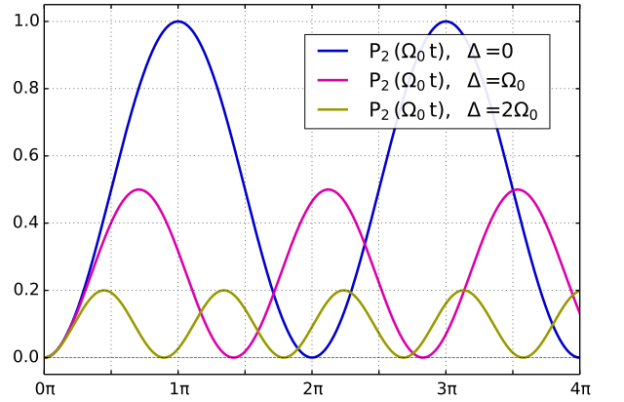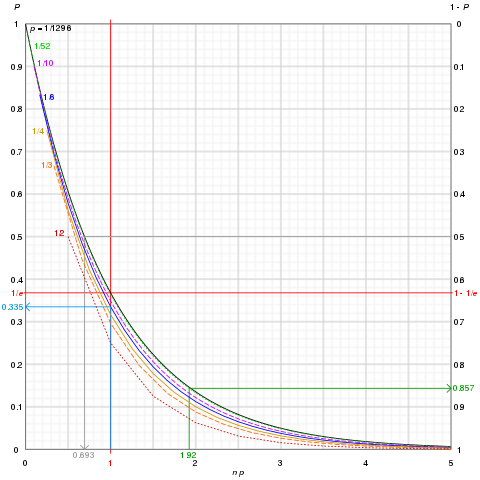Are you more of a visual learner? Check out our online video lectures and start your statistics course now for free!Image : “Mplwp Rabi oscillations” by

## Formula

In a case, denoted by k, possible outcomes are likely to occur as a result of an event. Each event will have a probability of 1/k. In case an event is denoted by “A,” the probability of any event occurring in a sample space “S” can be measured by the following formula:

P (A)= Count of outcomes in A / Count of outcomes in S
P (A)= Count of outcomes in A / S

## Bernoulli Trials

This is a situation that refers to two possible outcomes or results of a situation (success or failure). The probability of a successful outcome in the Bernoulli trial is denoted by ‘p.’ The successful outcome in this probability model is called a free throw, and failure is called a miss. The model states that each outcome is independent and does not affect other outcomes or results. Thus, one free throw or miss is unlikely to affect the probability associated with the next event.

Example: Suppose a coin is flipped again and again. Every time a single action of flipping a coin has occurred, it gives rise to equal results of “success” or “failure” that the head will appear. The factors involved in determining the probability of whether the head or tail will be shown on each flip are:

n = Number of trials

p = Probability of success in one trial

q = 1 – p = Probability of failure in one trial

k = Number of successes

n – k = Number of failures

Assuming the Bernoulli trials are independent, the outcome relies on the 10% rule.

The graph of Bernoulli trials will be shown as follows:Image: “Graphs of probability P of not observing independent events each of probability p after n trials vs n x p for various p. The graphs are actually discrete points since each n is an integer but drawn as continuous for clarity. 3 examples are shown: Blue arrow: Throwing a 6-sided die 6 times gives a 33.5% chance that 6 never turns up; as n increases, the probability that a 1/n-chance event never appears after n tries rapidly converges to 1/e. Grey arrow: To get a 50-50 chance of throwing a Yahtzee (5 cubic dice all showing the same number) requires 0.69 × 1296 ~ 898 throws. Green arrow: Drawing a card from a deck of playing cards without jokers 100 (1.92 × 52) times with replacement gives an 85.7% chance of drawing the ace of spades at least once.” by Cmglee – Own work. License: CC BY-SA 3.0

### The 10% Rule

The 10% rule in Bernoulli trials is interlined with the independence of outcomes from the probability of one event occurring. Free throw means that the event is independent and does not impact the probability of the next flipping of the coin.

Each time the coin is flipped, the expected outcome will be independent. In case it is not, the flipping activity can’t be continued on sample more than 10% of the total population. The 10% rule states that Bernoulli trials should be independent for the probability of each outcome. In case the trials depend on other outcomes, it should not proceed for more than 10% of the total population. This condition is applicable when the population is finite.

### Milgram experiment example

Stanley Milgram, a psychologist at Yale University, experimented with probability models in real life. He, the experimenter (E) ordered the teacher (T), who is also the subject of the tested experiment, to give an electric shock to a student (S) if he answers the question incorrectly each time.

Electric shocks used in the experiment were fake and the learner had to act as if he is getting an actual electric shock. Milgram experimented with it to check whether people obey authority if something contradicting with their personal conscience is required or not. He tested all probability models to find out the results, i.e., whether the teacher obeyed the order.

As only two outcomes were expected, the trial was called Bernoulli trials. Milgram called each person a single trial. Success meant the teacher obeyed the order to give the shock and failure meant refusal to obey. The Bernoulli trial model estimated a 65% success rate. The probability for a success rate came out P = 0.65.

### The geometric model: waiting for success

This model shows the probability of success and only success in a trial or event. In the case of coin flipping, the geometric model only counts head occurrence (success). The geometric distribution starts when success happens in a trial. It is applicable when there are multiple trials. The conditions for the geometric model involve independence and identical distribution of trials.

### Geometric probabilities

If n represents a number of independent trials, p indicates the probability of success and (1 − p) depicts the probability of failure, then:

P (success on the nth trial) = (1 − p) n−1 p

The variables involved in the geometric model:

P = Probability of success

X = Random variable related to success

If X=, then all attempts before the success will be denoted as .  So

P(X =) = (1-p) ƙ -1 P, for ƙ = 1, 2. . . . .

E[X] = 1/p

SD(X) = √1-p/p2

If X= Free number of throws, then the probability p = 0.60 using the above-mentioned equation.

### Milgram experiment example

The Milgram experiment provided the probability of success when the teacher agreed to give a shock to the learner who answered a question incorrectly. When the first teacher accepted the order, the trial resulted in first success resulting in:

The first teacher accepts

P (1st Teacher accepts) = 0.65

The third teacher accepts

The probability for success continues and provides a probability of success in geometric series. I.e.

P (1st refusal and 2nd refusal, 3rd acceptance) = 0.35 × 0.35 × 0.65

= 0.352 × 0.65 ≈ 0.08

The tenth teacher accepts

P (9 refusal, 10th acceptance) = 0.35 × · · · × S 0.35 × 0.65

9 Times

= 0.359 × 0.65

= 0.000512

### The binomial model: counting successes

The binomial probability model concerning discrete outcome referring to counting success or desired outcomes. In this model, the number of successes or “Y” is counted by finding out how many times the head will appear on flipping a coin for example. Suppose if we flip the coin 10 times, it will help us find the probability of success for 9 times (head appearance). Y, in this case, is the random variable referring to free throw or success.

Counting success

The number of successes, or free throws, have to be counted when n number of Bernoulli trials which have a “p” probability of occurrence of success “Y.” The determining factor is how many times the “Y” factor or success is probable.

## Combinations

It relates to counting success in the binomial model without writing out all possibilities, including misses or failures. It refers to a situation where n number of trials can give rise to results in n number of trials or attempts.

What is (n y) Analogy?

(n y) = n! / y! (n-y) in this equation, the exclamation mark refers “factorial.”

n! = n (n-1) (n-2). . . . . (2) (1).

The above-mentioned equation states that all integers have to be multiplied from n down to 1.

With the help of the given equation, we can find out the probabilities of success or head in coin-flipping. The trials have to be independent if we require a probability of py (1 – p) n – y

The above-mentioned equation states that all integers have to be multiplied from n down to 1.

(n y) ways of n number of expected success give the expected probability of heads in this way:

P(Y = y) = (n y)py(1 – p)n-y, for y = 1, 2, …, n

Using the binomial model, the probability can be measured in this equation:

E[Y] = np, SD(Y) = √np (1 – p)

## Normal approximation to Binomial Model

In case the number of species in a population increases, the sample size gets large, or the number of trials gets so large that the binomial model is unable to handle the related probabilities of success, approximate binomial probabilities can be used in this situation for desired results. Suppose a coin has to be flipped 500 times and you want the probability of heads more than 200 times. You can use Y as a binomial random variable with 500 trials having 0.6 probability of success. The equation will be as follows:

P(Y ≥ 400) = P(Y = 400) + P(Y = 401) + … + P(Y =500)

Now to find out the 101 probabilities by using the binomial model formula, it may take too long. The following formula can be used known as normal approximation taking Y as a binomial random variable with n number of trials and p as probability of success (occurrence of head); E[Y] = np, SD(Y) =. Standard deviation and mean can be used in the normal approximation of the binomial model. This model is useful in case success and failure only two outcomes are expected.

The condition for the application of a normal model for binomial probability is success/failure condition. In case the success/failure condition is duly satisfied, then mean and standard deviation can be calculated as “np”. In the above example where we have flipped the coin for 500 times and want to get head occurrence for 400 times, we want a probability of P(Y ≥ 400). Upon calculation through binomial formula, we may get a probability of 0.0022.

## The issue with the Normal Approximation

Normal approximation in the Binomial model can help in finding out the desired Y probability of success, but it has a big issue. It can’t be used to find out a particular number of successes or head occurrences. It can’t help out to find out the exact 400 head occurrence or free throws in case of a coin example.

As the random variable “Y” is involved in a normal approximation of a binomial model, it gives an equal chance to any value in a range to be chosen; hence, it is not possible to find out the exact possible outcomes of success.

Learn. Apply. Retain.
Your path to achieve medical excellence.
Study for medical school and boards with Lecturio.